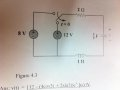# Step Response RCL Circuit

#### forbi

Joined Sep 11, 2012
37
Question : Find v(t) when t>0 .Before solving this question i have some doubts i need to clarify.

I been learning RC/RL Circuit and RCL Circuit.
So RC Circuit are classified as First Order and RCL Circuit are Second Order?

What it means Source Free RC/RL Circuit and Step Response RC/RL Circuit?
Does it means that at t<0 the Capacitor/Inductor is up and when t>0 it starts to decay without the source?
If my above analogy is correct, in order to know whether it is Source Free or Step Response Circuit, we just have to see if there is source attached to it when t>0?

Now to what i done so far for the question:
i got the steps from referencing my textbook.

At time = 0-
i(0+) = i(0-) = 8/2 = 4A ---- why i(0+) = i(0-)

v(0+) = v(0-) = 2i(0-) = 8V --- why v(0+) = v(0-)

α = R / 2L = 2/2 = 1
ω(o) = 1/ sqrt(LC) = 1 / sqrt [(1)(1/5)] = 2.236 -- what is this α and ω representing? Is the formula being use for all RCL Circuit analysis?

S(1,2) = -1 ± √[(1^2) - (2.236^2)] = -1 ± j2

Since α < ω(o) it is underdamped. ---- what is underdamped,critically damped and overdamped.

v(t) = (A1 cos ωt + A2 sin ωt) e ^(-αt) + Vs

dv/dt = -A1 + 2A2
dv(0)/dt = 4 / C = 20 ---- why we need get the dv/dt and dv(0)/dt to compare?

Now im stuck on how to find the V(0) .
when t<0 the voltage source supplied to the circuit is 8v and t>0 is 12v.
but i do not know how to find the voltage across the capacitor which is series with e voltage source, inductor and cap.

It is alot of question. But i hope someone could explain these to me as im very confused with this new stuff being taught to me.

#### WBahn

Joined Mar 31, 2012
29,130
Yes, an RC or RL circuit is first order and an RLC is second order.

I don't know what is meant by a "Source Free" circuit versus a "Step Response" circuit. Your author may be using their own terminology. I would need more context to hazzard a guess that I would have much confidence in.

You give a current at t=0. What current is that? It's not marked on the diagram. Don't make us guess.

If that current is flowing through the capacitor, then isn't the capacitor voltage changing? But the whole notion of the state at t=0- is that the switch has been in that position for long enough that the circuit has settled down and is not changing.

Why do you think that v(0-) is 0? Here I assume you are talking about the voltage across the capacitor since that voltage does appear to be marked on the diagram (hard to read, but I think that's a 'v').

To determine the initial conditions, place the switch in the configuration it is in just prior to t=0 and then analyze the circuit under the long-time steady state conditions in which all capacitors have no current in them and all inductors have no voltage across them (i.e., they look like opens and shorts respectively). Then carry across those things that can't change instantly (capacitor voltage and inductor current) as you close the switch and move from t=0- to t=0+.